• 基数排序时间复杂度
2021-12-16 09:15:36

对于给定的r个d位数，取值范围为[0,n]，我们使用计数排序比较元素的每一位，基数排序耗时Θ(r+n)，那么基数排序的复杂度为Θ(d(r+n))。排序
更多相关内容
• 基数排序：先通过他们的个位数进行排序，再把个位排好的放到新数组中，再对他们的十位数进行排序（此时排完个位数都放在同一区域内并且个位数已经排好），再对他们的百位进行排序，一直排到对数组里最大的的位数进行...

基数排序：先通过他们的个位数进行排序，再把个位排好的放到新数组中，再对他们的十位数进行排序（此时排完个位数都放在同一区域内并且个位数已经排好），再对他们的百位进行排序，一直排到对数组里最大的的位数进行排序。排完最大位数取到新数组时排序完成。

那怎么分别把它们通过位数进行排序呢？我们定义一个二维数组，把它叫做桶，通过把位数大小进行区分放在不同二维数组内，如下图：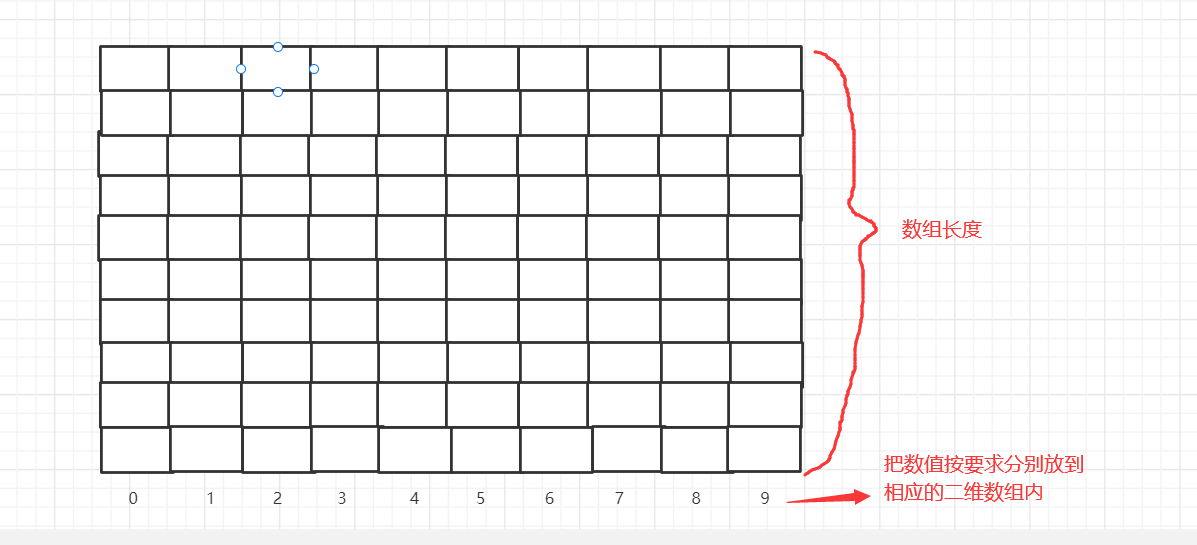我们以此数组为例：arr={12,5,66,156,378,43,1987,250,544,109} 对他进行基数排序

1）先把个位数按照数字大小进行排序分别放入桶内，如下图：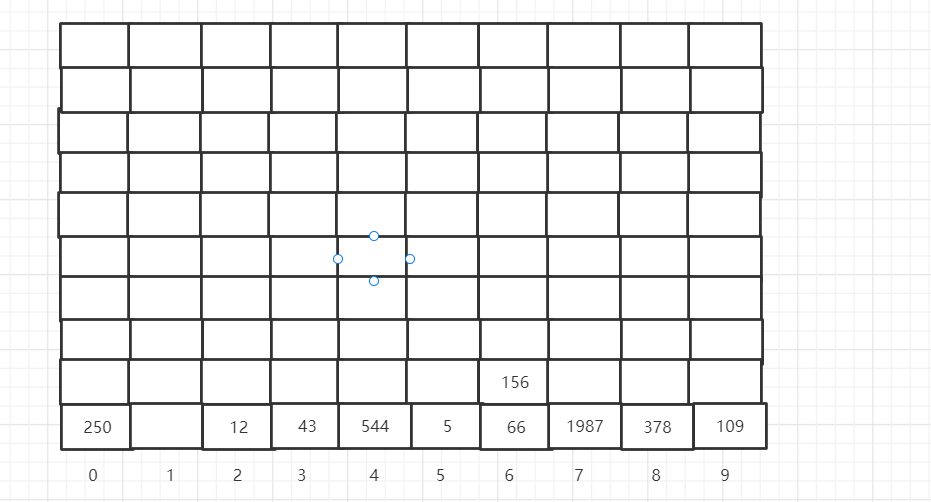2）再把它们按在桶内顺序从小到大从桶放入数组arr内 ，如下图：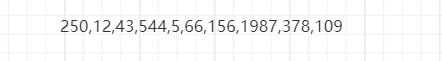3） 在对十位按照数字大小进行排序分别放入桶内（此时个位已经排好都放在0中，我的例子个位数只有一个所以看不出来），如下图：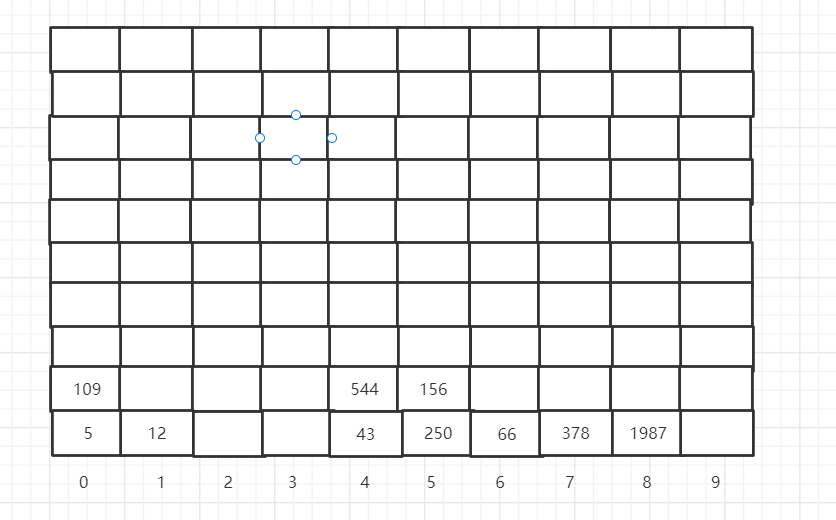4）再把它们按桶内顺序放进数组内，如下图：5）  再对百位按照数字大小进行排序分别放入桶内（很明显此时十位已经排好都放在二维数组0中），如下图：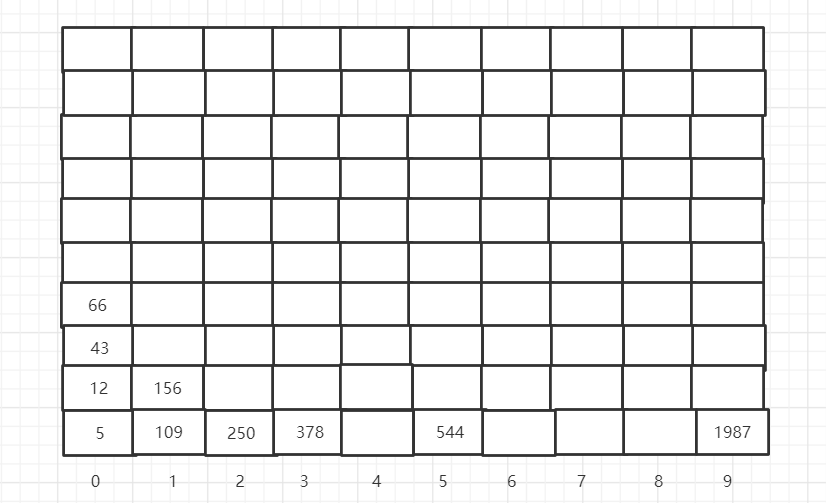6）重复上述步骤，放入数组内，如下图：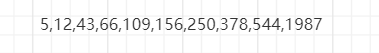7）排千位放入桶中，重复上述步骤：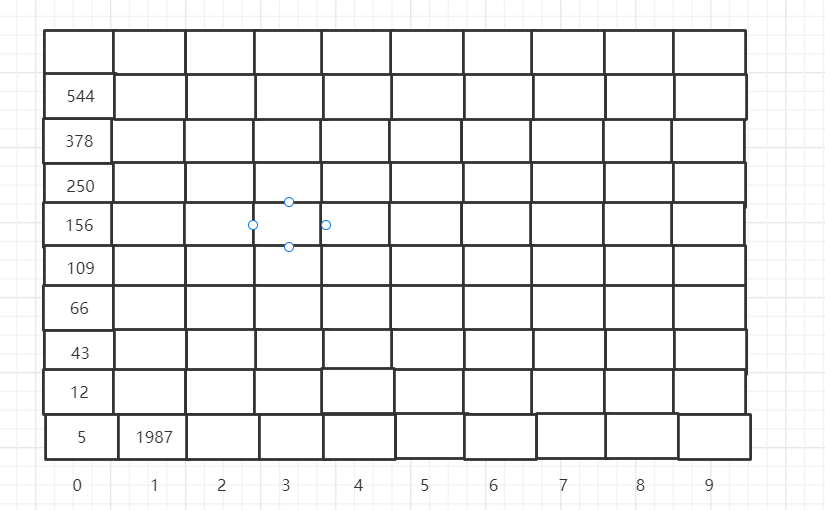8）此时排序已经结束，放入数组内，排序完成：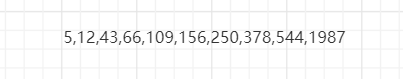实现代码如下：

public class Jssort {
public static void main(String[] args) {
//定义要排序的二维数组
int[] arr= {12,3,111,32,556,3321,789,567,22213,43};
//调用排序方法
sort(arr);
//输出拍完序的数组
System.out.println(Arrays.toString(arr));
}

private static void sort(int[] arr) {
//定义数组第一个数默认为最大值
int max=arr;
//对数组进行遍历找到最大值，来确认要放入桶中的次数
for(int i=1;i<arr.length;i++) {
if(max<arr[i]) {
max=arr[i];
}
}
//通过加空字符串的方法来获取最大值的位数
int maxindex=(max+"").length();
//定义默认位数，此时1是个位
int s=1;
//有几位数遍历几次
for(int i=0;i<maxindex;i++) {
//定义二维数组桶，第一个是桶宽默认10从0-9，第二个是桶高默认为数组的长度
int[][] buk=new int[arr.length];
//二维数组要知道一维数组每个分别放了几位数，下面定义的是记录每位放了几个数
int[] eCount=new int[arr.length];
//放入桶中
for(int j=0;j<arr.length;j++) {
//找到要排序位数的大小，s=1时是个位，s=10时是十位
int a=arr[j]/s%10;
//通过大小分别放入二维数组内
buk[a][eCount[a]]=arr[j];
//记录加一，再放大小相等的数时，有记录就不会放到别的数组内
eCount[a]++;
}
//定义新数组索引值
int index=0;
//把桶中数据按位数大小按顺序放入新数组中
for(int m=0;m<10;m++) {
//判断桶中是否有元素，没有就进入下一个桶
if(eCount[m]!=0) {
//放入一维数组中
for(int n=0;n<eCount[m];n++) {
arr[index]=buk[m][n];
index++;
}
}
//把记录置空，方便位数增加后记录数据
eCount[m]=0;
}
//排序结束，增加位数
s*=10;
}

}
}

结果如下图：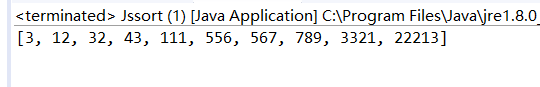时间复杂度：O（k*n）,最外层时间复杂度是o（k）,k是序列最大数的位数，在里层的出桶时间复杂度是数组的长度O(n)，再拼接成数组的时间复杂度平均是数组的长度O（n）,总的来说，基数排序的时间复杂度是o(k*(n+n)),即O（k*n）

适用于位数不多，每一位范围不大的序列。

展开全文java 排序算法
• 基数排序的思想 基数排序基数排序的思想是把位数相同的一组数组...注意：基数排序每次位的比较可以使用线性排序的方式，比如桶排序或者计数排序，因为它们的时间复杂度为O(n)，而且每轮的比较需要保证每次比较数...

### 基数排序的思想

基数排序，基数排序的思想是把位数相同的一组数组依次从后往前比较其每一位上的大小，经过几轮比较使得数据达到有序的做法。比较的次数跟数据的位数有关系。比如要比较一组手机号码从小到大排列，可以比较手机号每一位大小，然后比较11次，手机号达到有序。

注意：基数排序每次位的比较可以使用线性排序的方式，比如桶排序或者计数排序，因为它们的时间复杂度为O(n)，而且每轮的比较需要保证每次比较数据的稳定性，不然基数排序就无法完成。

### 图解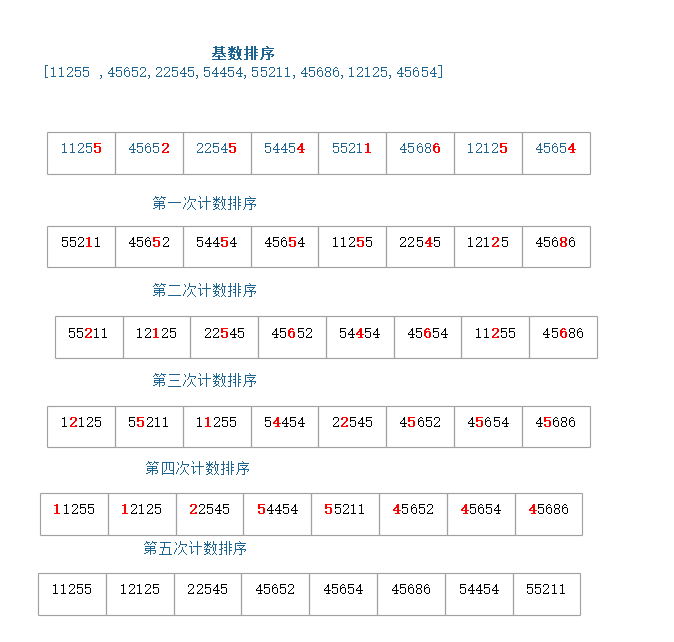### 代码

package com.study.algorithm.sort;

public class RadixSort {

/**
* 基数排序
*
* @param arr
*/
public static void radixSort(int[] arr) {
int max = arr;
for (int i = 0; i < arr.length; i++) {
if (arr[i] > max) {
max = arr[i];
}
}

// 从个位开始，对数组arr按"指数"进行排序
for (int exp = 1; max / exp > 0; exp *= 10) {
countingSort(arr, exp);
}
}

/**
* 计数排序-对数组按照"某个位数"进行排序
*
* @param arr
* @param exp 指数
*/
public static void countingSort(int[] arr, int exp) {
if (arr.length <= 1) {
return;
}

// 计算每个元素(位0~9)出现的个数
int[] c = new int;
for (int i = 0; i < arr.length; i++) {
c[(arr[i] / exp) % 10]++;
}

// 计算排序后的位置
for (int i = 1; i < c.length; i++) {
c[i] += c[i - 1];
}

// 临时数组r，存储排序之后的结果
int[] r = new int[arr.length];
for (int i = arr.length - 1; i >= 0; i--) {
r[c[(arr[i] / exp) % 10] - 1] = arr[i];
c[(arr[i] / exp) % 10]--;
}

for (int i = 0; i < arr.length; i++) {
arr[i] = r[i];
}
}
public static void printArr(int[] arr,int n){
for (int i = 0; i < n ; i++) {
System.out.print("["+arr[i]+"]");
}
}
public static void main(String[] args) {
int[] arr={11255 ,45652,22545,54454,55211,45686,12125,45654};
printArr(arr,8);

}
}

### 基数排序的时间复杂度

基数排序每一位的比较可以使用线性排序，比如桶排序或者计数排序，当然需要保证如计数排序的稳定性。每次排序时间复杂度O(n)，那么如果有k位，则时间复杂度为O(k*n),如果k不大比如手机号11位，那么时间复杂度就是O(n)

基数排序是稳定排序算法

### 基数排序是原地排序算法吗？

基数排序基于线性排序如计数排序，所以不是原地排序算法。

### 基数排序的应用场景

基数排序对数据有要求，要能够将数据分割成独立的位。每一位的数据范围不能太大，要能够使用线性排序来实现。基数排序数据的长度要相等，不等的话需要补齐。

展开全文基数排序 排序算法
• 算法思想：计数排序无需比较关键字，而是通过分配和收集来实现排序，时间复杂度为线性阶O（n）。对于十进制数来说，每一位在0~9之间，d位的数，则有d列。基数排序首先按低位哟有效数字排，然后逐位向上一位排，直到...

算法思想：计数排序无需比较关键字，而是通过分配和收集来实现排序，时间复杂度为线性阶O（n）。对于十进制数来说，每一位在0~9之间，d位的数，则有d列。基数排序首先按低位哟有效数字排，然后逐位向上一位排，直到高位排序结束。

约定：待排数据中没有0和负数，如果有负数转为正数即可。

public class 基数排序 {
//pos=1表示个位，pos=2表示十位
public static int getNumInPos(int num, int pos) {
int tmp = 1;
for (int i = 0; i < pos - 1; i++) {
tmp *= 10;
}
return (num / tmp) % 10;
}
//求得最大位数d
public static int getMaxWeishu(int[] a) {
int max = a;
for (int i = 0; i < a.length; i++) {
if (a[i] > max)
max = a[i];
}
int tmp = 1, d = 1;
while (true) {
tmp *= 10;
if (max / tmp != 0) {
d++;
} else
break;
}
return d;
}
public static void radixSort(int[] a, int d) {
int[][] array = new int[a.length + 1];
for (int i = 0; i < 10; i++) {
array[i] = 0;
// array[i]记录第i行数据的个数
}
for (int pos = 1; pos <= d; pos++) {
for (int i = 0; i < a.length; i++) {
// 分配过程
int row = getNumInPos(a[i], pos);
int col = ++array[row];
array[row][col] = a[i];
}
for (int row = 0, i = 0; row < 10; row++) {
// 收集过程
for (int col = 1; col <= array[row]; col++) {
a[i++] = array[row][col];
}
array[row] = 0;
// 复位，下一个pos时还需使用
}
}
}
public static void main(String[] args) {
int[] a = { 49, 38, 65, 197, 76, 213, 27, 50 };
for (int i : a)
System.out.print(i + " ");
}
}

展开全文排序算法
• 对于数组中所有的元素，利用元素每一位的值进行排序，如十进制元素数组[342,254,87]，则先对个位排序，再对十位排序，最后对百位排序。由于十进制每一位范围为0-9，因此按位排序的过程调用计数排序。示意图图下： 2...
• 基数排序（radix sort）属于“分配式排序”（distribution sort），又称“桶子法”（bucket ...其时间复杂度为O (nlog(r)m)，其中r为所采取的基数，而m为堆数，在某些时候，基数排序法的效率高于其它的稳定性排序法。排序算法 基数排序 计数排序
• 本文我们将继续介绍一种线性时间复杂度的排序算法--基数排序，这种排序算法的时间复杂度为Θ(d(n+k))，这种排序基于我们之前将的计数排序，其中n表示待排序列的规模，d表示待排序列的最大位数，k表示每一位数的范围...
• 什么是基数排序？ （一）基数排序的思想：把待排序的整数按位分，分为个位，十位.....从小到大依次将位数进行排序。实际上分为两个 过程：分配和收集。  分配就是：从个位开始，按位数从小到大把数据排好，分别...图片
• 文章目录一、写在前言二、排序（一）基数排序1、思路讲解2、代码实现三、排序算法时间复杂度比较四、结束语 一、写在前言 我哭了，写的博客没有保存，睡了一觉之后电脑不知道怎么关机了。啊啊啊啊蓝瘦想哭，木有心情...排序算法 快速排序 数据结构
• 时间复杂度 最优：O(nlog(n)) 最差：O(n^2) 平均：O(nlog(n))合并排序合并排序是一种分治算法。这个算法不断地将一个数组分为两部分，分别对左子数组和右子数组排序，然后将两个数组合并为新的有序数组。 稳定：...归并排序 快速排序
• 时间复杂度 平均情况 时间复杂度 最好情况 时间复杂度 最坏情况 空间复杂度 插入排序 是 O(n²) O O(n²) O 希尔排序 （Shell） 是...排序算法
• 桶、计数、基数排序算法中，除了桶排序在桶粒度大于1时要通过比较排序外，其他两种排序都不需要使用比较就能使数列完成排序，这也是为什么时间复杂度可以达到线性O(n)的原因。 桶排序 桶排序的求解思路为找出数列...桶排序 排序算法 计数排序 基数排序
• 时间复杂度达到O(n)的不同于sort给予比较的O(nlogn)排序，是基于计数的一种线性排序方法，效率非常优秀。
• 排序算法-算法时间复杂度和空间复杂度概念 详细讲解 排序算法的介绍 排序也称排序算法(Sort Algorithm)，排序是将一组数据，依指定的顺序进行排列的过程。 排序的分类： 1)内部排序： 指将需要处理的所有数据都加载...java
• 冒泡排序的名字是根据排序算法的特性得出的，每一个元素，像一个气泡，从最初的起始位置，一步步冒到最终位置。 冒泡每次交换相邻的两个元素，（假设是从小到大排序），每一轮冒泡，起码可以把最大的元素，从最初...排序算法
• 常用的排序算法的时间复杂度和空间复杂度 排序法 最差时间分析 平均时间复杂度 稳定度 空间复杂度 冒泡排序 O(n2) O(n2) 稳定 O(1) 快速排序 O(n2) O(nlog2n) 不稳定 O(log2n)~O(n) 选择排序 O(n2)
• 内排序有可以分为以下几类：（1）插入排序：直接插入排序、二分法插入排序、希尔排序（2）选择排序：直接选择排序、堆排序（3）交换排序：冒泡排序、快速排序（4）归并排序（5）基数排序排序方法时间复杂度(平均)...
• 分类：比较排序、非比较排序 比较排序：通过元素间的比较进行排序，时间复杂度不能超过O(nlogn)，不在乎数据规模和分布情况。...包括：基数排序、计数排序、桶排序。 算法复杂度 排序方法 时间复杂度(平...go
• 时间复杂度 空间复杂度       稳定性           关联性        最好          最差        平均  ...排序算法 数据结构
• //联系人:石虎QQ:1224614774昵称:嗡嘛呢叭咪哄 ...排序图表: 一、插入排序 　每次将一个待排序的数据，跟前面已经有序的序列的数字一一比较找到自己合适的位置，插入到序列中，直到全部数据插入完成。 二...快排的原理 稳定性 排序算法
• 1.冒泡排序 冒泡排序的基本原理 对存放原始数组的数据，按照从前往后的方向进行多次扫描，每次扫描...冒泡排序时间复杂度：O（n^2） O(N)和真实的计算时间成正比 从前到后执行一轮要n次，O(N) N指的是数据的规模.数据结构
• 1.排序算法的介绍 排序也称排序算法(Sort Algorithm)，排序是将一组数据，依指定的顺序进行排列的过程。 2.排序算法的分类 内部排序: ...3. 算法的时间复杂度 3.1 度量一个程序(算法)执行时间...数据结构 java 排序算法
• 各种排序算法比较各种常用排序算法类别排序方法时间复杂度空间复杂度稳定性复杂性特点最好平均最坏辅助存储简单插入排序直接插入O(N)O(N2)O(N2)O(1)稳定简单希尔排序O(N)O(N1.3)O(N2)O(1)不稳定复杂选择排序直接选择...js排序的时间复杂度
• 基数排序是一种非比较型整数排序算法，其原理是将众多数字按位分隔后进行排序。 实现步骤： 1.将所有待比较的数字（正整数）统一为同一长度，位数不够的数字前面补0； 2.按照从个位，十位，百位······从低到高...
• 文章目录一、排序算法的介绍二、排序的分类三、算法的时间复杂度3.1 度量一个程序(算法)执行时间的两种方法3.2 时间频度3.3 时间复杂度3.4 常见时间复杂度3.5 平均时间复杂度和最坏时间复杂度四、算法的空间复杂度 ...排序算法
• 基数排序(桶排序)介绍: (1)基数排序(radix sort)属于"分配式排序"(distribution sort),又称"桶子法"(bucket sort)或bin sort,顾名思义,它是通过键值的各个位的值,将要排序的元素分配至某些"桶"中,达到排序的作用 ...数据结构
• 排序算法的时间复杂度和空间复杂度 &amp;amp;nbsp; 其中冒泡排序加个标志，所以最好情况下是o(n) &amp;amp;nbsp;直接选择排序排序过程： &amp;amp;nbsp;&amp;amp;nbsp;&amp;amp;nbsp;...
• 算法的时间复杂度与初始序列无关的是：选择排序、堆排序、归并排序、基数排序 算法的排序趟数与初始序列无关的是：插入排序、选择排序、基数排序 堆排序 (1)堆是一颗完全二叉树； (2)小(大)顶堆中的每一个节点都不...堆排序 数据结构 排序算法 快速排序
• 2、 基数排序时间复杂度为O（N*M），其中N为数据个数，M为数据位数 二、 辅助记忆 1、时间复杂度记忆 冒泡、选择、直接 排序需要两个for循环，每次只关注一个元素，平均时间复杂度为O（n * n）O（n * n）（一遍找...排序算法 空间复杂度 稳定性...